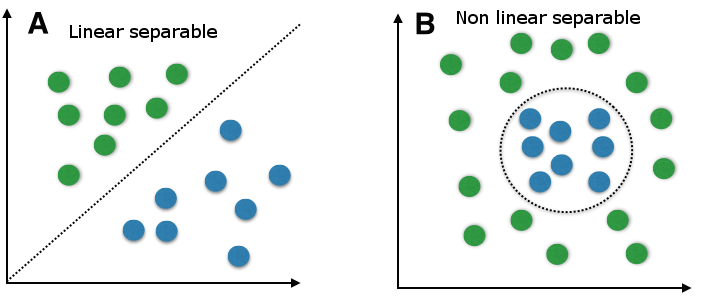# Support Vector Machine

By Learnbay Category Machine Learning Reading time 9-12 mins Published on Nov 25, 2020

## Introduction of Support Vector Machine

Support vector machines (SVMs) are a particularly powerful and flexible class of supervised algorithms for both classification and regression.

SVMs were introduced initially in the 1960s and were later refined in 1990s. However, it is only now that they are becoming extremely popular, owing to their ability to achieve brilliant results. SVMs are implemented uniquely when compared to other Machine Learning algorithms.

Support vector machine(SVM) is a supervised learning algorithm that is used to classify the data into different classes, now unlike most algorithms SVM makes use of hyperplane which acts as a decision boundary between the various classes. In general, SVM can be used to generate multiple separating the hyperplane so that the data is divided into segments. These segments contain some kind of data. SVM used to classify the data into two different segments depending on the feature of data.

### Feature of Support Vector Machine SVM-

SVM studies the labeled data & then classify any new input data depending on what it learned into the training phase.

It can be used for both classification and regression problems. As SVC supports vector classification SVR stands for support vector regression. One of the main features of SVM is kernel function, it can be used for nonlinear data by using the kernel trick. The working of the kernel trick is to transform the data into another dimension so that we can draw a hyperplane that classifies the data.

How SVM work??

SVM works by mapping data to a high-dimensional feature space so that data points can be classified, even when the data are not linearly separable. A separator between the classifies is found, then the data are transformed in such a way that the separator could be drawn as a hyperplane. Following this, characteristics of new data can be used to predict the group to which a new record should belong.

Importing Libraries:

import pandas as pd

import numpy as np

import matplotlib.pyplot as plt

%matplotlib inline

Exploratory Data Analysis:

bankdata.shape

 Variance Skewness Curtosis Entropy Class 0 3.62160 8.6661 -2.8073 -0.44699 0 1 4.54590 8.1674 -2.4586 -1.46210 0 2 3.86600 -2.6383 1.9242 0.10645 0 3 3.45660 9.5228 -4.0112 -3.59440 0 4 0.32924 -4.4552 4.5718 -0.98880 0

Data preprocessing:

X = bankdata.drop('Class', axis=1)

y = bankdata['Class'] from sklearn.model_selection import train_test_split

X_train, X_test, y_train, y_test = train_test_split(X, y, test_size = 0.20)

Training the Algorithm:

from sklearn.svm import SVC

svclassifier = SVC(kernel='linear')

svclassifier.fit(X_train, y_train)

Making prediction

y_pred = svclassifier.predict(X_test)

Evaluating the Algorithm:

from sklearn.metrics import classification_report, confusion_matrix

print(confusion_matrix(y_test,y_pred))

print(classification_report(y_test,y_pred))

Output:

[[152 0] [ 1 122]] precision recall f1-score support

0 0.99 1.00 1.00 152

1 1.00 0.99 1.00 123

avg / total 1.00 1.00 1.00 275

### SVM Linear Classifier:

In the linear classifier model, we assumed that training examples plotted in space. These data points are expected to be separated by an apparent gap. It predicts a straight hyperplane dividing 2 classes. The primary focus while drawing the hyperplane is on maximizing the distance from hyperplane to the nearest data point of either class. The drawn hyperplane called a maximum-margin hyperplane.

### SVM Non-Linear Classifier:

In the real world, our dataset is generally dispersed up to some extent. To solve this problem separation of data into different classes based on a straight linear hyperplane can’t be considered a good choice. For this Vapnik suggested creating Non-Linear Classifiers by applying the kernel trick to maximum-margin hyperplanes. In Non-Linear SVM Classification, data points plotted in a higher-dimensional space.Learnbay provides industry accredited data science courses in Bangalore. We understand the conjugation of technology in the field of Data science hence we offer significant courses like Machine Learning, Tensor Flow, IBM Watson, Google Cloud platform, Tableau, Hadoop, time series, R and Python. With authentic real-time industry projects. Students will be efficient by being certified by IBM.